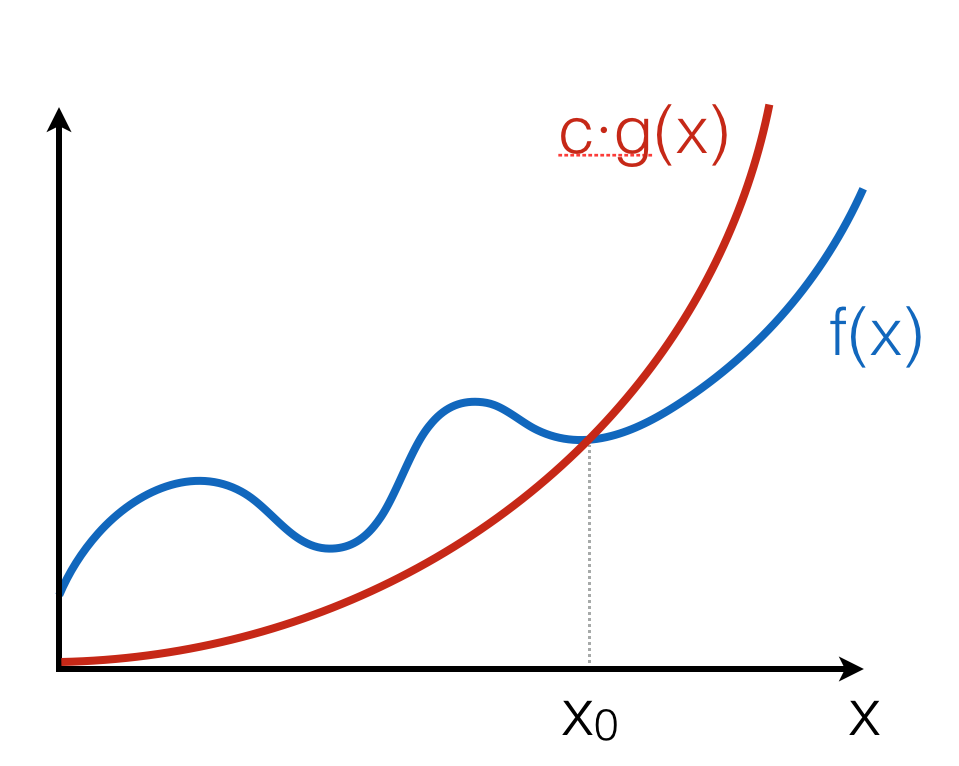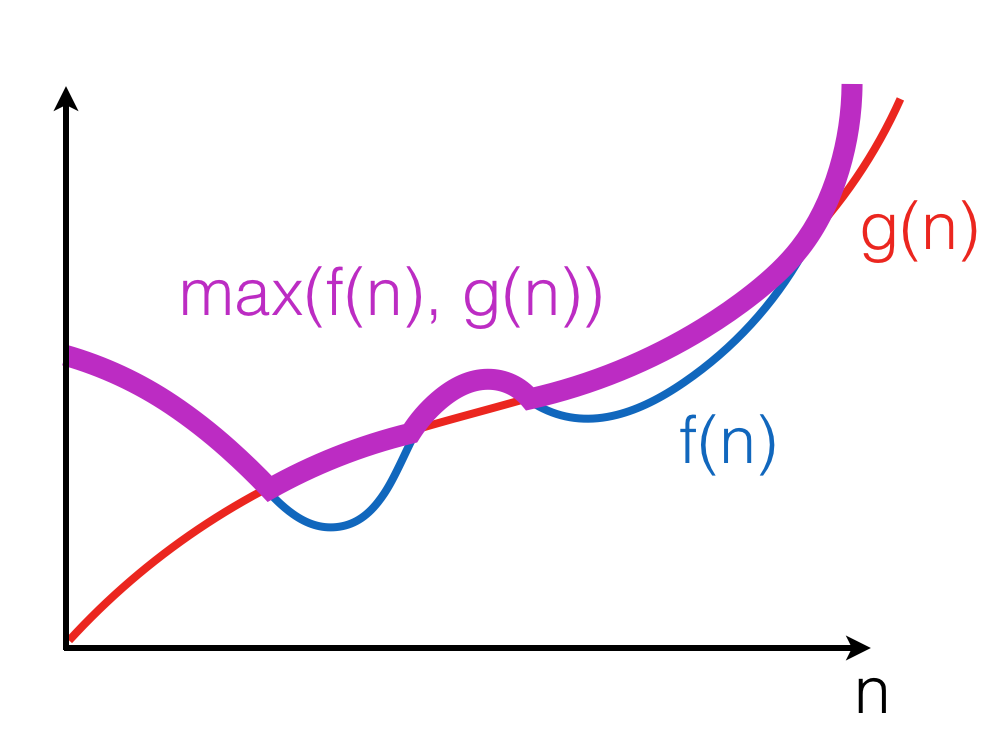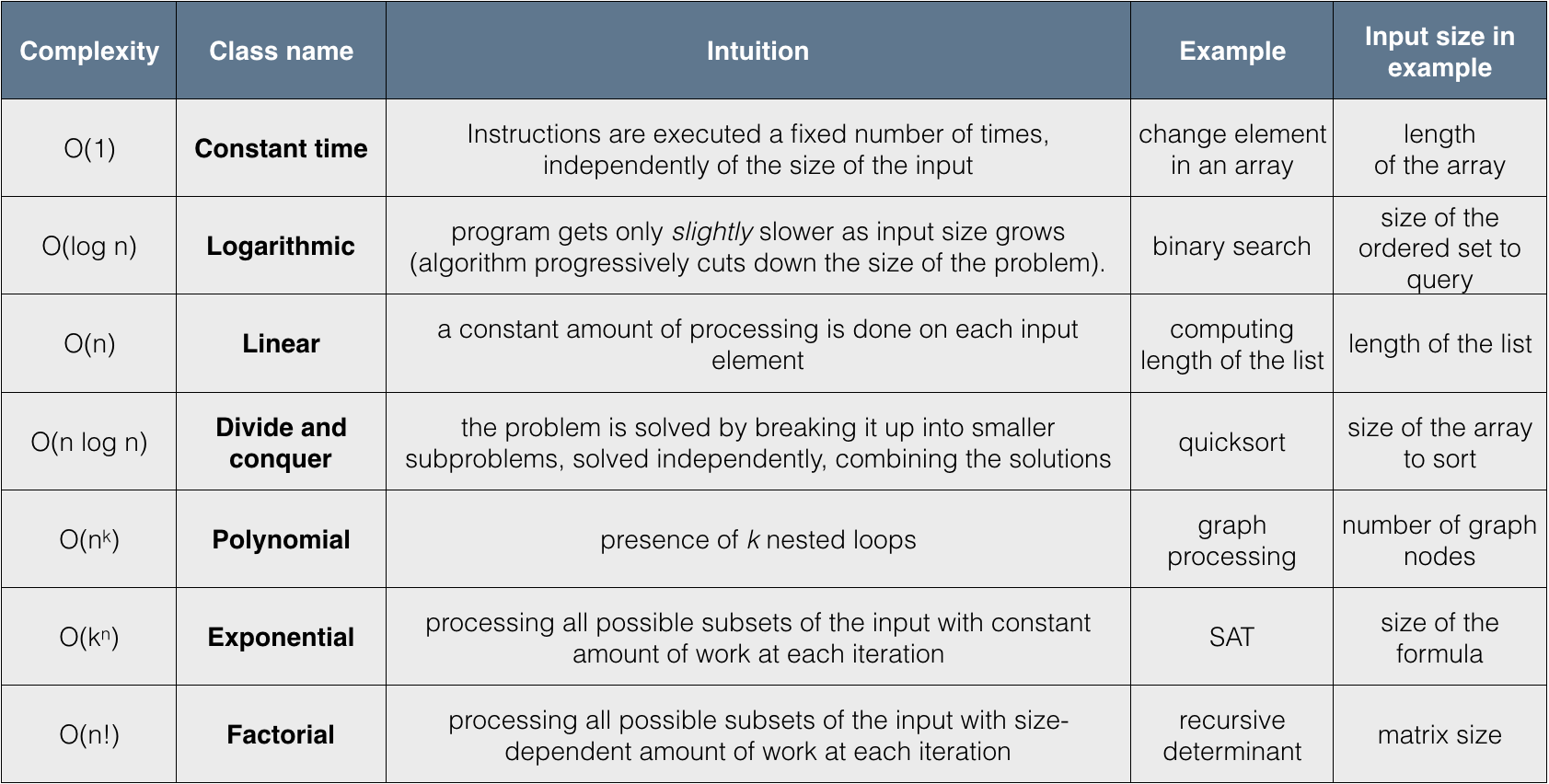# 3.3. Order Notation¶

Let us introduce a concise notation for asymptotic behaviour of time demand functions as an input size $$n$$ of an algorithm grows infinitely, i.e., $$n \rightarrow \infty$$.

## 3.3.1. Big O-notation¶

Definition

The positive-valued function $$f(x) \in O(g(x))$$ if and only if there is a value $$x_0$$ and a constant $$c > 0$$, such that for all $$x \geq x_0$$, $$f(x) \leq c \cdot g(x)$$.

This definition can be illustrated as follows:The intuition is that $$f(x)$$ grows no faster than $$c \cdot g(x)$$ as $$x$$ gets larger. Notice that the notation $$O(g(x))$$ describes a set of functions, “approximated” by $$g(x)$$, modulo constant factors and the starting point.

## 3.3.2. Properties of Big O-notation¶

Property 1

$$O(k \cdot f(n)) = O(f(n))$$, for any constant $$k$$.

Multiplying by $$k$$ just means re-adjusting the values of the arbitrary constant factor $$c$$ in the definition of big-O. This property ensures machine-independence (i.e., we can forget about constant factors). Since $$\log_{a}n = \log_{a}b \times \log_{b}n$$, we don’t need to be specific about the base when saying $$O(\log~n)$$.

Property 2

$$f(n) + g(n) \in O(\max(f(n), g(n))$$

Here, $$\max((f(n), g(n))$$ is a function that for any n, returns the maximum of $$f(n)$$ and $$g(n))$$:The property follows from the fact that for any $$n, f(n) + g(n) \leq 2 \cdot f(n)$$ or $$f(n) + g(n) \leq 2 \cdot g(n)$$. Therefore, $$f(n) + g(n) \leq 2 \cdot \max(f(n), g(n))$$.

Property 3

$$\max(f(n), g(n)) \in O(f(n) + g(n))$$.

This property follows from the fact that for any $$n, \max(f(n), g(n)) \leq f(n)$$ or $$\max(f(n), g(n)) \leq g(n)$$. Therefore, $$\max(f(n), g(n)) \leq f(n) + g(n)$$.

Corollary

$$O(f(n) + g(n)) = O(\max(f(n), g(n))$$.

Property 4

If $$f(n) \in O(g(n))$$, then $$f(n) + g(n) \in O(g(n))$$.

Follows from the fact that there exist $$c, n_0$$, such that for any $$n \geq n_0, f(n) \leq c \cdot g(n)$$; Therefore, for any $$n \geq n_0, f(n) + g(n) \leq (c + 1) \cdot g(n)$$. Intuitively, a faster-growing function eventually dominates.

## 3.3.3. Little o-notation¶

Definition

The positive-valued function $$f(x) \in o(g(x))$$ if and only if for all constants $$\varepsilon > 0$$ there exists a value $$x_0$$ such that for all $$x \geq x_0, f(x) \leq \varepsilon \cdot g(x)$$.

This definition provides a tighter boundary on $$f(x)$$: it states that $$g(x)$$ grows much faster (i.e., more than a constant factor times faster) than $$f(x)$$.

Example

We can show that $$x^2 \in o(x^3)$$, as for any $$\varepsilon > 0$$ we can take $$x_0(\varepsilon) = \frac{1}{\varepsilon} + 1$$, so for all $$x \geq x_0(\varepsilon), \varepsilon \cdot x^3 \geq \varepsilon \cdot (\frac{1}{\varepsilon} + 1) \cdot x^2 > x^2$$.

## 3.3.4. Proofs using O-notation¶

Standard exercise: show that $$f(x) \in O(g(x))$$ (or not) is approached as follows:

• Unfold the definition of O-notation;
• Assuming that the statement is true, try to find a fixed pair of values $$c$$ and $$x_0$$ from the definition to prove that the inequality holds for any $$x$$;
• If such fixed pair cannot be found, as it depends on the value of $$x$$, then the universal quantification over $$x$$ in the definition doesn’t hold, hence $$f(x) \notin O(g(x))$$.

Example 1: Is $$n^2 \in O(n^3)$$?

Assume this holds for some $$c$$ and $$n_0$$, then:

\begin{split}\begin{align*} & n^2 - c \cdot n^3 \leq 0,~\text{for all}~n \geq n_0 \\ \implies & n^2 (1 - c \cdot n) \leq 0,~\text{for all}~n \geq n_0 \\ \implies & 1 \leq c \cdot n,~\text{for all}~n \geq n_0 \\ \implies & n \geq \frac{1}{c},~\text{for all}~n \geq n_0 \\ \end{align*}\end{split}

As this clearly holds for $$n_0 = 2$$ and $$c = 1$$, we may conclude that $$n^2 \in O(n^3)$$.

$$\square$$

Example 2: Is $$n^3 \in O(n^2)$$?

Assume this holds for some $$c$$ and $$n_0$$, then:

\begin{split}\begin{align*} & n^3 - c \cdot n^2 \leq 0,~\text{for all}~n \geq n_0 \\ \implies & n^2 \cdot (n - c) \leq 0,~\text{for all}~n \geq n_0 \\ \implies & n - c \leq 0,~\text{for all}~n \geq n_0 \\ \end{align*}\end{split}

Now, since $$c$$ and $$n_0$$ are arbitrary, but fixed, we can consider $$n = c + 1 + n_0$$ (and so we can do for any $$c$$ and $$n_0$$), so we see that the inequality doesn’t hold, hence in this case no fixed $$c$$ and $$n_0$$ can be found to satisfy it for any $$n$$. Therefore $$n^3 \notin O(n^2)$$.

$$\square$$

## 3.3.5. Hierarchy of algorithm complexities¶## 3.3.6. Complexity of sequential composition¶

Consider the following OCaml program, where a is a value of size n:

let x = f1(a)

in  x + f2(a)


Assuming the complexity of f1 is $$f(n)$$ and the complexity of f2 is $$g(n)$$, executing both of them sequentially leads to summing up their complexity, which is over-approximated by $$O(\max(f(n), g(n))$$. This process of “collapsing” big O’s can be repeated for a finite number of steps, when it does not depend on the input size.